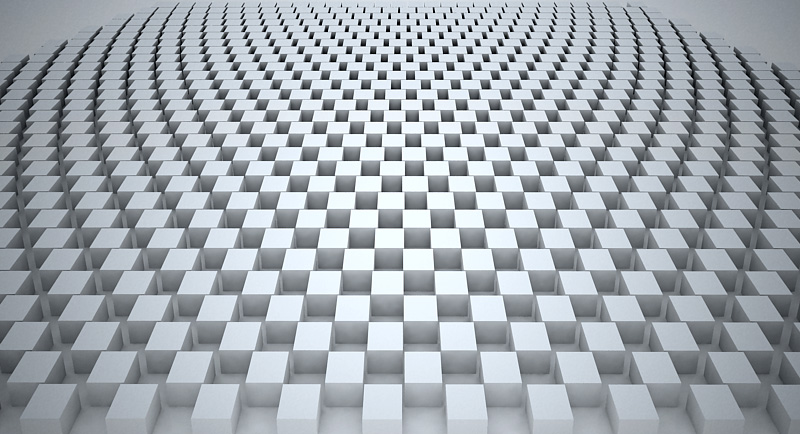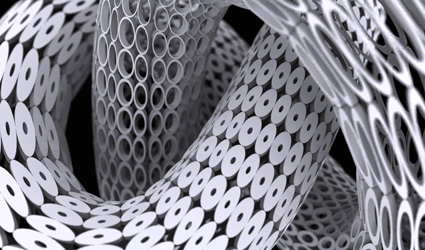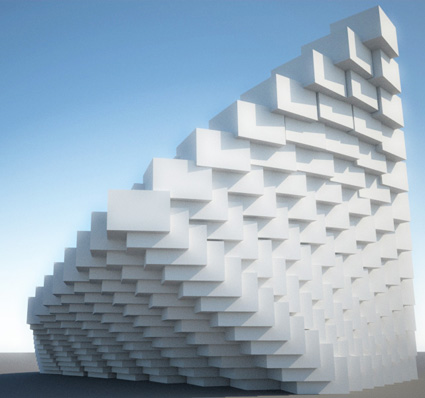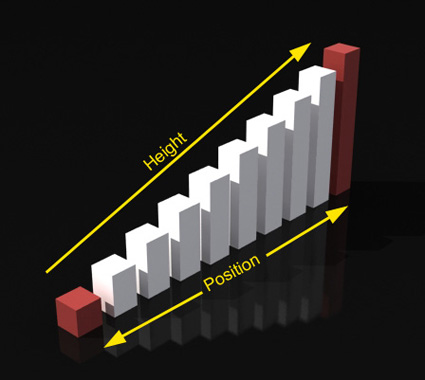# Ali Torabi

Digital Architecture & Technology

## Lineare controller inside script controller part 2

In This Video:

• How to control items along one direction using linear controller in a multi-dimensional array.
• Reference: linear controller function takes the following arguments
i: index value — index of item in X direction
j: index value — index of item in Y direction
K: index value — index of item in Z direction
dim: dimensions in point3 format — defines the directions that linear controller will be applied on.
for example [1,0,0] means only in x direction ,[0,1,1] means in Y and Z.
Note1: if you don’t pass the i,j or kargument to the function it considers the current item indexes by default.
Note2: if you dont pass the dim argument to the function it considers all directions by default.

, , , ,
April 18, 2010 at 10:14 am Comments (0)

## Linear transformation function and script controllerIn This Video:

• using linear controller inside the script controller.
• use conditional statement to set index value.
• set index1 if index2 is even number (running bond pattern).
• use i argument in linearcontroller function to change the current index.
• , , , , , , , ,
April 18, 2010 at 10:04 am Comments (2)

## Add noise effect using random controller.Level: Intermediate
In This Video:

• How to combine random controller and other controllers to get noise effect.
• Learn how to make a parametric lattice using mesh smooth and edit poly modifiers
• Update random values by pressing preview button
• , , , , , , , , , ,
April 17, 2010 at 5:29 pm Comments (0)

## Why preview!?Level: Intermediate
In This Video:

• Using preview option in complex and large arrays.
• How to update array counts while using preview mode.
• Array on mesh object using UV mapping data.
• , , , , , ,
April 17, 2010 at 5:16 pm Comments (2)

## Twisting louversIn This Video:

• Use curve controller to transform the gizmo box.
• How to twist objects using Vol select and Xform modifiers.
• , , , , , , ,
April 17, 2010 at 12:31 pm Comments (0)

## Parametric three dimensional arrayLevel: Intermediate
In This Video:

• Make Three dimensional array and use the linear controller to transform the objects.
• Learn how to pick the corners.
• , , , , ,
April 17, 2010 at 11:38 am Comment (1)

## Linear controller ,Incremental and total option

Level: Beginner
In This Video:

• Add items in between (Total) or at the end of array (Incremental).
• , , , , , ,
April 17, 2010 at 11:24 am Comments (14)

## Make your first linear arrayLevel: Beginner
In This Video:

• Make a simple live array. control the number of items and distance.
• Using linear controller.
• control the scalar property of objects (height) inside the array.
• , , , ,
April 17, 2010 at 11:02 am Comments (4)